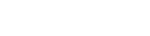# Random Numbers

Godot has a number of Random Number functions. The sequence of numbers that are generated are deterministic so each time you run your game, the same sequence of numbers is generated.

To change the sequence, but still have it be deterministic, you can pass an integer value to the `seed(int value)` function.

Another way is to use the `rand_seed()` function. This returns an array containing a 32bit signed integer random number and the next seed value. But there are easier functions to use than this.

For getting different random number sequences on each run, apply the `randomize()` function.

`float rand_range( float from, float to )` gives a floating point value between the specified limits.

`randf()` returns a float between 0 and 1. This may be used to flip between choices e.g.

``````if randf() > 0.5 print("A") else print("B")
``````

`randi()` returns a random unsigned 32-bit integer. To limit the range we may use the remainder from modulo division.

``````randi() % 8 # returns a number between 0 and 7
randi() % 100 # returns a number between 0 and 99
10 + randi() % 21 # returns a number between 10 and 30
``````

To more intuitively write the last example we could write `int(round(rand_range(10, 30)))`.

However, this functionality is built into the `RandomNumberGenerator` class.

``````var rng = RandomNumberGenerator.new()
print(rng.randi_range(-10, 10))
``````

To obtain a random number following a normal distribution:

``````var rng = RandomNumberGenerator.new()
rng.randomize()
print(rng.randfn())
``````

## Accessing array keys

The array size may be used as follows: `the_array[randi() % the_array.size()]`

We may use a shuffle bag approach where we remove a chosen element from the array to avoid it being picked again. Then, refill the array after it is empty.

Another method is picking based on weighted averages. Here we may assign weight values to an array of the same size as our data array. We add up these values to give a sum. Then get a random value between zero and sum. Then scan the array of weights, adding each one until the value exceeds our random value. Thus, we have the index value to select our random pick from our data array.

``````var data = ["a", "b", "c", "d"]
var weights = [1, 3, 4, 8]
var sum

sum = 0
for n in weights:
sum += n
print(pick())
print(pick())
print(pick())

func pick():
var p = randi() % sum
var w = 0
var idx = 0
for n in weights:
w += n
if w > p:
break
idx += 1
return data[idx]
``````

See the docs for more examples.